# ANALYTIC-GEOMETRY(4).pptx

15. Feb 2023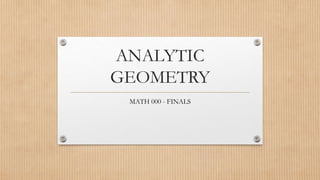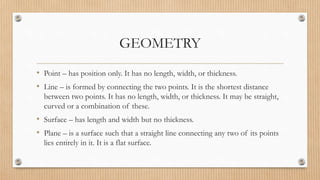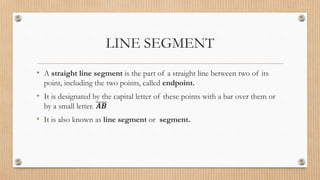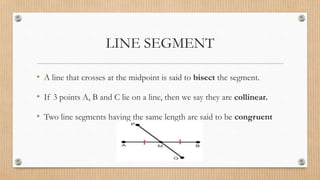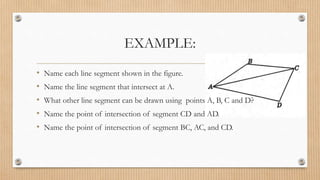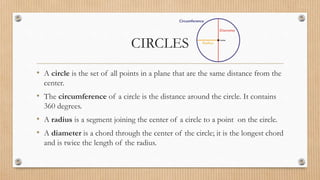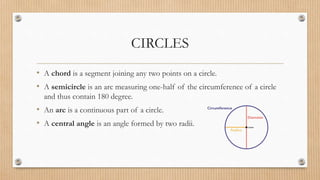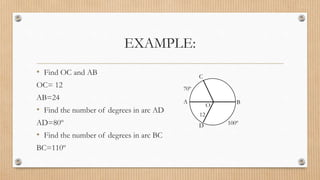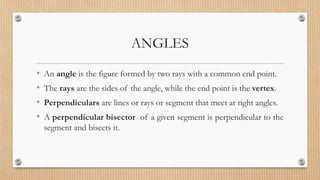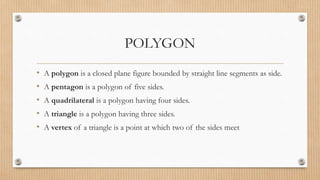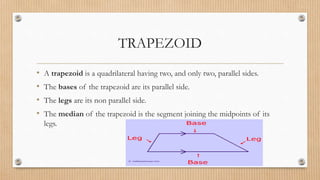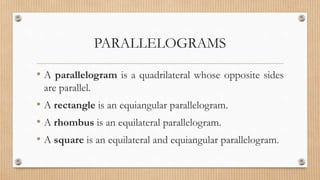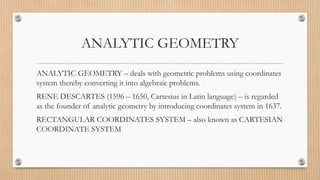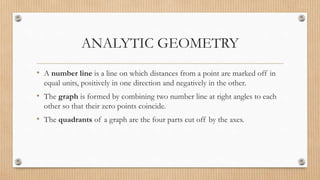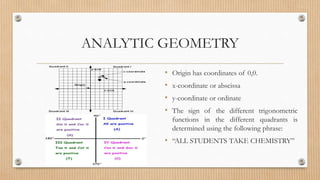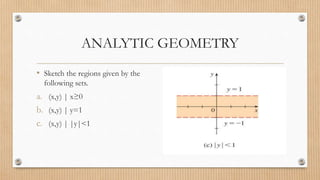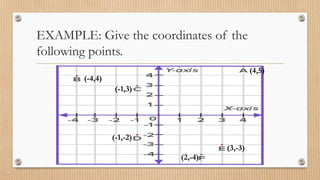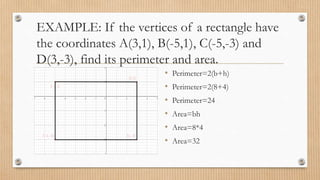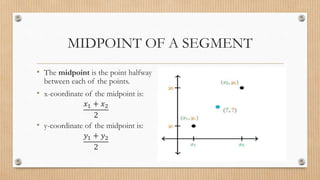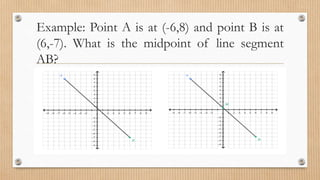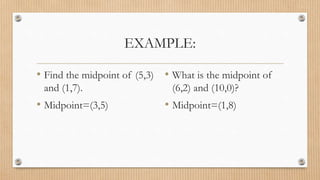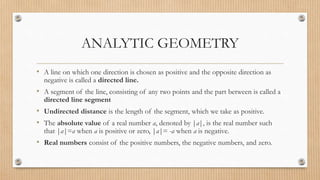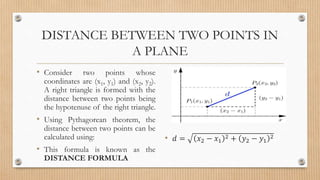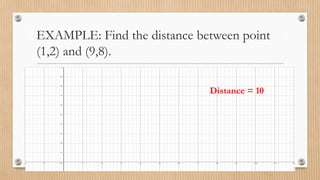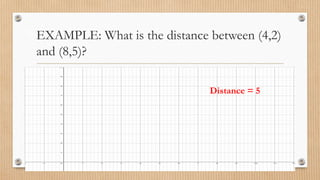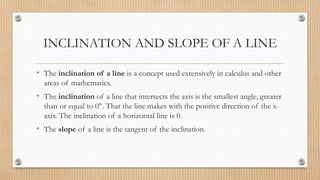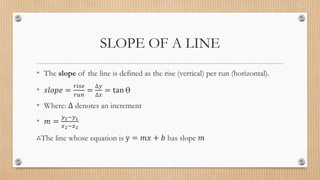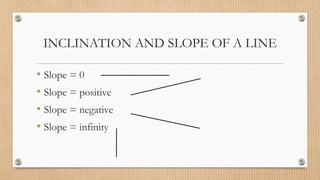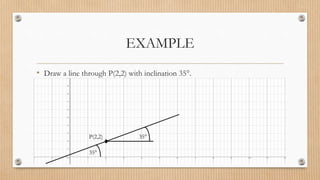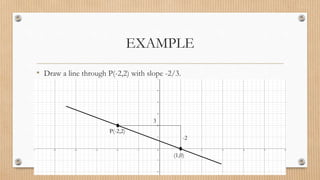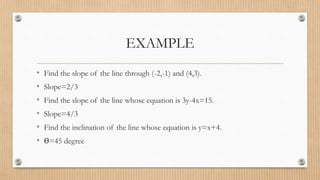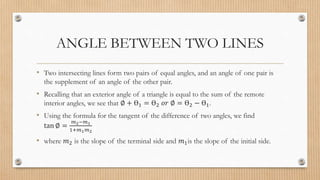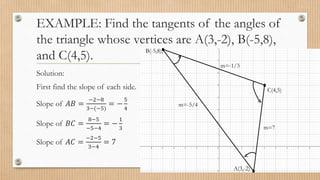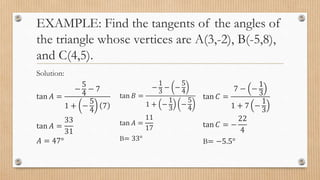1 von 34

### ANALYTIC-GEOMETRY(4).pptx

• 2. GEOMETRY • Point – has position only. It has no length, width, or thickness. • Line – is formed by connecting the two points. It is the shortest distance between two points. It has no length, width, or thickness. It may be straight, curved or a combination of these. • Surface – has length and width but no thickness. • Plane – is a surface such that a straight line connecting any two of its points lies entirely in it. It is a flat surface.
• 3. LINE SEGMENT • A straight line segment is the part of a straight line between two of its point, including the two points, called endpoint. • It is designated by the capital letter of these points with a bar over them or by a small letter. 𝑨𝑩 • It is also known as line segment or segment.
• 4. LINE SEGMENT • A line that crosses at the midpoint is said to bisect the segment. • If 3 points A, B and C lie on a line, then we say they are collinear. • Two line segments having the same length are said to be congruent
• 5. EXAMPLE: • Name each line segment shown in the figure. • Name the line segment that intersect at A. • What other line segment can be drawn using points A, B, C and D? • Name the point of intersection of segment CD and AD. • Name the point of intersection of segment BC, AC, and CD.
• 6. CIRCLES • A circle is the set of all points in a plane that are the same distance from the center. • The circumference of a circle is the distance around the circle. It contains 360 degrees. • A radius is a segment joining the center of a circle to a point on the circle. • A diameter is a chord through the center of the circle; it is the longest chord and is twice the length of the radius.
• 7. CIRCLES • A chord is a segment joining any two points on a circle. • A semicircle is an arc measuring one-half of the circumference of a circle and thus contain 180 degree. • An arc is a continuous part of a circle. • A central angle is an angle formed by two radii.
• 8. EXAMPLE: • Find OC and AB OC= 12 AB=24 • Find the number of degrees in arc AD AD=80º • Find the number of degrees in arc BC BC=110º C D A B 70º 100º 12 O
• 9. ANGLES • An angle is the figure formed by two rays with a common end point. • The rays are the sides of the angle, while the end point is the vertex. • Perpendiculars are lines or rays or segment that meet at right angles. • A perpendicular bisector of a given segment is perpendicular to the segment and bisects it.
• 10. POLYGON • A polygon is a closed plane figure bounded by straight line segments as side. • A pentagon is a polygon of five sides. • A quadrilateral is a polygon having four sides. • A triangle is a polygon having three sides. • A vertex of a triangle is a point at which two of the sides meet
• 11. TRAPEZOID • A trapezoid is a quadrilateral having two, and only two, parallel sides. • The bases of the trapezoid are its parallel side. • The legs are its non parallel side. • The median of the trapezoid is the segment joining the midpoints of its legs.
• 12. PARALLELOGRAMS • A parallelogram is a quadrilateral whose opposite sides are parallel. • A rectangle is an equiangular parallelogram. • A rhombus is an equilateral parallelogram. • A square is an equilateral and equiangular parallelogram.
• 13. ANALYTIC GEOMETRY ANALYTIC GEOMETRY – deals with geometric problems using coordinates system thereby converting it into algebraic problems. RENE DESCARTES (1596 – 1650, Cartesius in Latin language) – is regarded as the founder of analytic geometry by introducing coordinates system in 1637. RECTANGULAR COORDINATES SYSTEM – also known as CARTESIAN COORDINATE SYSTEM
• 14. ANALYTIC GEOMETRY • A number line is a line on which distances from a point are marked off in equal units, positively in one direction and negatively in the other. • The graph is formed by combining two number line at right angles to each other so that their zero points coincide. • The quadrants of a graph are the four parts cut off by the axes.
• 15. ANALYTIC GEOMETRY • Origin has coordinates of 0,0. • x-coordinate or abscissa • y-coordinate or ordinate • The sign of the different trigonometric functions in the different quadrants is determined using the following phrase: • “ALL STUDENTS TAKE CHEMISTRY”
• 16. ANALYTIC GEOMETRY • Sketch the regions given by the following sets. a. (x,y) | x≥0 b. (x,y) | y=1 c. (x,y) | |y|<1
• 17. EXAMPLE: Give the coordinates of the following points. (4,5) (-4,4) (-1,3) (-1,-2) (3,-3) (2,-4)
• 18. EXAMPLE: If the vertices of a rectangle have the coordinates A(3,1), B(-5,1), C(-5,-3) and D(3,-3), find its perimeter and area. • Perimeter=2(b+h) • Perimeter=2(8+4) • Perimeter=24 • Area=bh • Area=8*4 • Area=32
• 19. MIDPOINT OF A SEGMENT • The midpoint is the point halfway between each of the points. • x-coordinate of the midpoint is: 𝑥1 + 𝑥2 2 • y-coordinate of the midpoint is: 𝑦1 + 𝑦2 2
• 20. Example: Point A is at (-6,8) and point B is at (6,-7). What is the midpoint of line segment AB?
• 21. EXAMPLE: • Find the midpoint of (5,3) and (1,7). • Midpoint=(3,5) • What is the midpoint of (6,2) and (10,0)? • Midpoint=(1,8)
• 22. ANALYTIC GEOMETRY • A line on which one direction is chosen as positive and the opposite direction as negative is called a directed line. • A segment of the line, consisting of any two points and the part between is called a directed line segment • Undirected distance is the length of the segment, which we take as positive. • The absolute value of a real number a, denoted by |a|, is the real number such that |a|=a when a is positive or zero, |a|= -a when a is negative. • Real numbers consist of the positive numbers, the negative numbers, and zero.
• 23. DISTANCE BETWEEN TWO POINTS IN A PLANE • Consider two points whose coordinates are (x1, y1) and (x2, y2). A right triangle is formed with the distance between two points being the hypotenuse of the right triangle. • Using Pythagorean theorem, the distance between two points can be calculated using: • This formula is known as the DISTANCE FORMULA • 𝑑 = 𝑥2 − 𝑥1 2 + 𝑦2 − 𝑦1 2
• 24. EXAMPLE: Find the distance between point (1,2) and (9,8). Distance = 10
• 25. EXAMPLE: What is the distance between (4,2) and (8,5)? Distance = 5
• 26. INCLINATION AND SLOPE OF A LINE • The inclination of a line is a concept used extensively in calculus and other areas of mathematics. • The inclination of a line that intersects the axis is the smallest angle, greater than or equal to 0°. That the line makes with the positive direction of the x- axis. The inclination of a horizontal line is 0. • The slope of a line is the tangent of the inclination.
• 27. SLOPE OF A LINE • The slope of the line is defined as the rise (vertical) per run (horizontal). • 𝑠𝑙𝑜𝑝𝑒 = 𝑟𝑖𝑠𝑒 𝑟𝑢𝑛 = ∆𝑦 ∆𝑥 = tan Ɵ • Where: ∆ denotes an increment • 𝑚 = 𝑦2−𝑦1 𝑥2−𝑥2 ∴The line whose equation is y = 𝑚𝑥 + 𝑏 has slope 𝑚
• 28. INCLINATION AND SLOPE OF A LINE • Slope = 0 • Slope = positive • Slope = negative • Slope = infinity
• 29. EXAMPLE • Draw a line through P(2,2) with inclination 35°. P(2,2) 35° 35°
• 30. EXAMPLE • Draw a line through P(-2,2) with slope -2/3. P(-2,2) -2 3 (1,0)
• 31. EXAMPLE • Find the slope of the line through (-2,-1) and (4,3). • Slope=2/3 • Find the slope of the line whose equation is 3y-4x=15. • Slope=4/3 • Find the inclination of the line whose equation is y=x+4. • Ɵ=45 degree
• 32. ANGLE BETWEEN TWO LINES • Two intersecting lines form two pairs of equal angles, and an angle of one pair is the supplement of an angle of the other pair. • Recalling that an exterior angle of a triangle is equal to the sum of the remote interior angles, we see that ∅ + Ɵ1 = Ɵ2 𝑜𝑟 ∅ = Ɵ2 − Ɵ1. • Using the formula for the tangent of the difference of two angles, we find tan ∅ = 𝑚2−𝑚1 1+𝑚1𝑚2 • where 𝑚2 is the slope of the terminal side and 𝑚1is the slope of the initial side.
• 33. EXAMPLE: Find the tangents of the angles of the triangle whose vertices are A(3,-2), B(-5,8), and C(4,5). Solution: First find the slope of each side. Slope of 𝐴𝐵 = −2−8 3−(−5) = − 5 4 Slope of 𝐵𝐶 = 8−5 −5−4 = − 1 3 Slope of 𝐴𝐶 = −2−5 3−4 = 7 A(3,-2) B(-5,8), C(4,5) m=-1/3 m=-5/4 m=7
• 34. EXAMPLE: Find the tangents of the angles of the triangle whose vertices are A(3,-2), B(-5,8), and C(4,5). Solution: tan 𝐴 = − 5 4 − 7 1 + − 5 4 7 tan 𝐴 = 33 31 𝐴 = 47° tan 𝐵 = − 1 3 − − 5 4 1 + − 1 3 − 5 4 tan 𝐴 = 11 17 B= 33° tan 𝐶 = 7 − − 1 3 1 + 7 − 1 3 tan 𝐶 = − 22 4 B= −5.5°